## Forecasts with the Polynomial Regression Model in Excel

Regression analysis aims to model the expected values for a dependent variable (y) based on independent variables (x).

The polynomial regression is a statistical technique to fit a non-linear equation to a data set by employing polynomial functions of the independent variable. We can use the model whenever we notice a non-linear relationship between the dependent and independent variables.

The first publication on the polynomial regression originated in the early 19th century. The model played an essential role in the development of regression analysis through the 20th century.

## What is the polynomial regression model?

A polynomial model is a form of regression analysis. We use an N-th degree polynomial to model the relationship between the dependent variable y and the predictor x.

The goal is to fit a non-linear model to the relationship between dependent and independent variables. However, as a statistical problem, the polynomial equation is linear in terms of the parameters we estimate from the data set.

We consider the model to be a specific case of multiple linear regression. If we have more than one independent variable, we can create a combined variable to use. For example, if we have the predictors xa and xb, we can use x1 = xa*xb for our polynomial regression.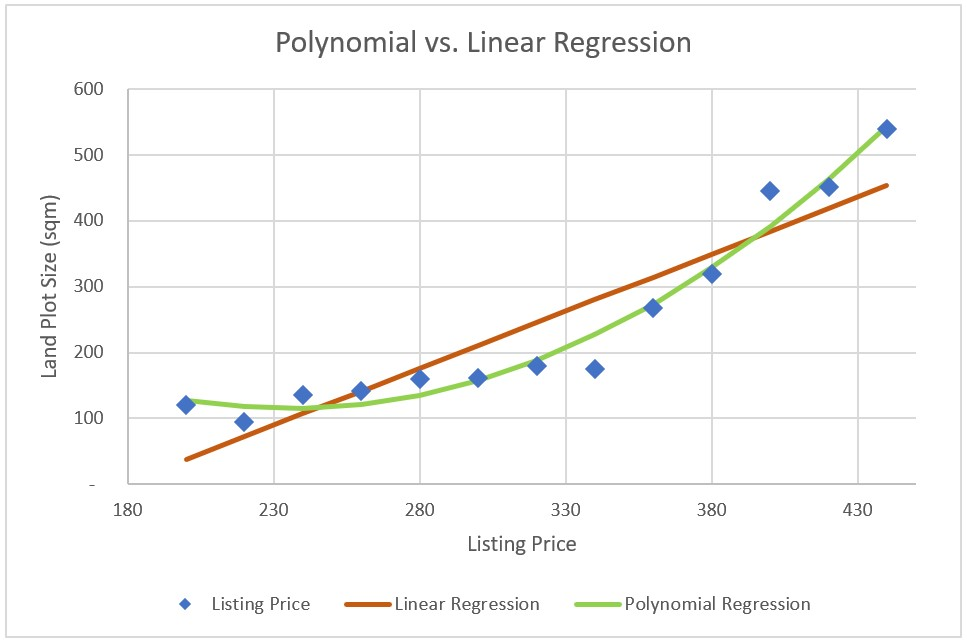If we look at a single linear regression equation, we can represent it with the following function:This equation keeps a linear yield increase, meaning for each added unit of x1, we get precisely β1 units added to y.

More often than not, such linear relationships won’t work in reality. For example, if we model the yield in conversion rate based on marketing spending, it may turn out that the marginal yield per unit increases with a more significant spend. One way to approach such a case would be with a quadratic polynomial equation.The change in yield/unit depends on x, and this is why the model is non-linear, even if it is linear in terms of the parameters we are estimating.

If we model the expected value for y as an N-th degree (N-order) polynomial, we can use the general polynomial regression model that we denote like this: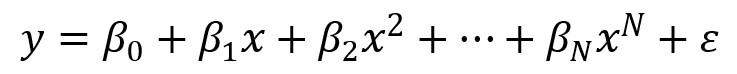These models are linear from the perspective of estimation because the function is linear in terms of the parameters (e.g., β0, β1).

## And Get a FREE Benchmark Analysis Template

### Polynomial Orders (Degrees)

A first degree (N = 1) polynomial regression is essentially a simple linear regression with the function: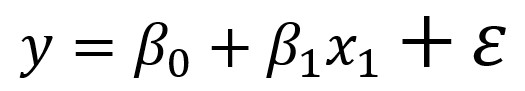A 2nd order polynomial represents a quadratic equation with a parabolic curve and a 3rd-degree one – a cubic equation.

The polynomial equationas a polynomial is the same as the multiple regressionWhere:### Polynomial Model Principles

To be reliable, the polynomial regression needs a large number of observations in the data set.

We must also remember not to extrapolate the observed values further than the available time series and base our regression model only on the available data.

As for statistical metrics, we can use the p-values to support our model, but only if the plot looks reasonable.

Large predictor (x) values can cause issues with the model, so we may scale it down to have smaller, more manageable values.

Polynomial regression analysis is most suitable for highly controlled environments, and it can often show illogical results for some potions of the fitted curve. We must always apply common sense to our models to ensure we have a good plot.

### Assumptions

When we use polynomial regression with a data set, we need to consider the model’s inherent limitations, resulting from the underlying assumptions.

The target variable (y) follows an additive relation between itself and a set of N independent variables (xi; i = 1 to N).

The relationship between the target value and any predictor (independent variable) has to be linear or curvilinear, and the predictors have to be independent of each other.

The residual errors follow a normal distribution with a mean of zero and a constant variance (OLS).

### Fitting the model

We fit the polynomial regression model by employing the Least Squares Method.

Generally, more degrees in our model will tend to result in better performance of the model. However, it is crucial to remember that if we try to fit polynomials of a too high degree, we may overfit our model.

We can find the right degree (or order) by increasing it to the point we see enough significance to define the best possible model (called forward selection). We can also do the reverse by decreasing the degree (backward selection).

### Interpretation

The polynomial regression is a multiple linear regression from a technical point of view. However, we do not interpret it the same way.

It is often quite challenging to look at individual coefficients, as the underlying predictors (independent variables) in a polynomial regression model may experience a strong correlation. It is more insightful to look at the fitted regression function as a whole. Then we can use confidence bands to mitigate any uncertainty within the model.

The main disadvantage of the polynomial models is their sensitivity to outliers in the data set. Even a single outlier can significantly impact the results and render our analysis useless.

The main problem is that there are not many tools and techniques to detect outliers in non-linear regression models.

## Example

To illustrate the polynomial regression model, let’s look at the following data set. We have 20 observations of marketing campaigns, with the marketing spend in euro and the achieved conversion rate in percentage points.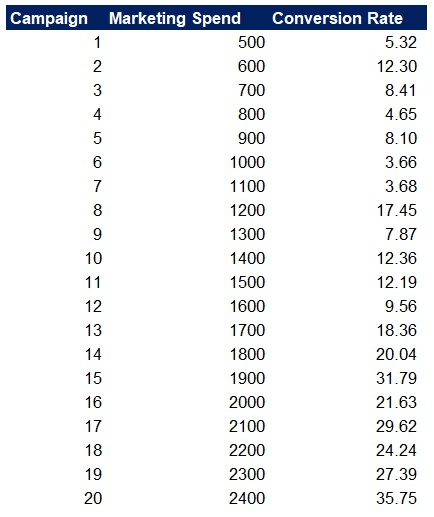We are looking to fit an equation to the data set that we can use to estimate the conversion rate based on marketing spend. If we plot the observations on a scatter diagram in Excel, we get the following. We can also add a trendline, and let’s pick a linear one for now.

You can add a trendline with a right-click on the data points and selecting Trendline. To learn more about trendlines and linear regression, please refer to our dedicated article on the matter.Apart from showing the equation and the R-squared on the chart, we can also calculate the linear regression slope and intercept with the respective Excel functions, SLOPE, and INTERCEPT.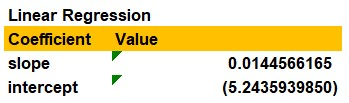We can then use these and the linear regression equation to estimate the conversion rate for five additional marketing spend levels.And here is our chart with the estimated values. We can see they fall on the Trendline, as we used its equation for the calculation.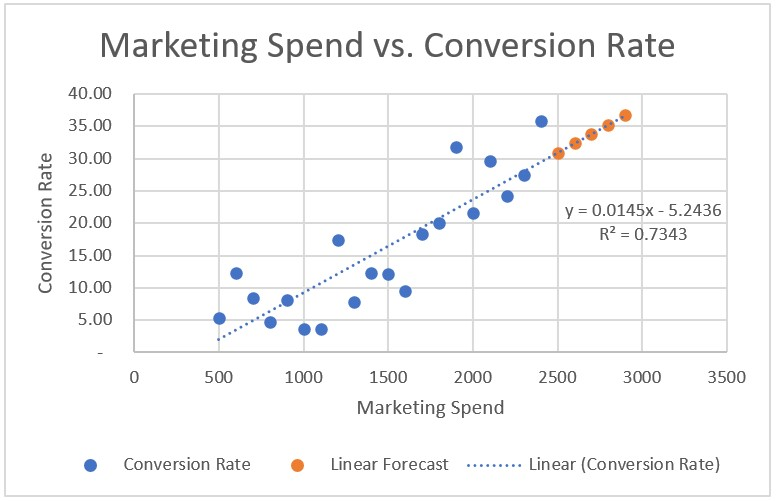However, it seems like the growth of the conversion rate accelerates more than the increased marketing spends. Let’s change our Trendline to a Polynomial one with an Order of two.

As the coefficients are small, the equation showing on the chart is not very useful, so that we will calculate the coefficients on our own. We can also notice that the R-squared is 0.81 compared to 0.73 on our linear regression, indicating a better fit to the data set.Using a combination of the INDEX and LINEST functions in Excel, we can calculate the three coefficients for the 2nd-degree polynomial equation. You can take a closer look at the formulas in the example Excel file.Using these and the polynomial equation, we can calculate estimated values for our model. Ensure that the b2 coefficient is multiplied by the independent variable to the power of two.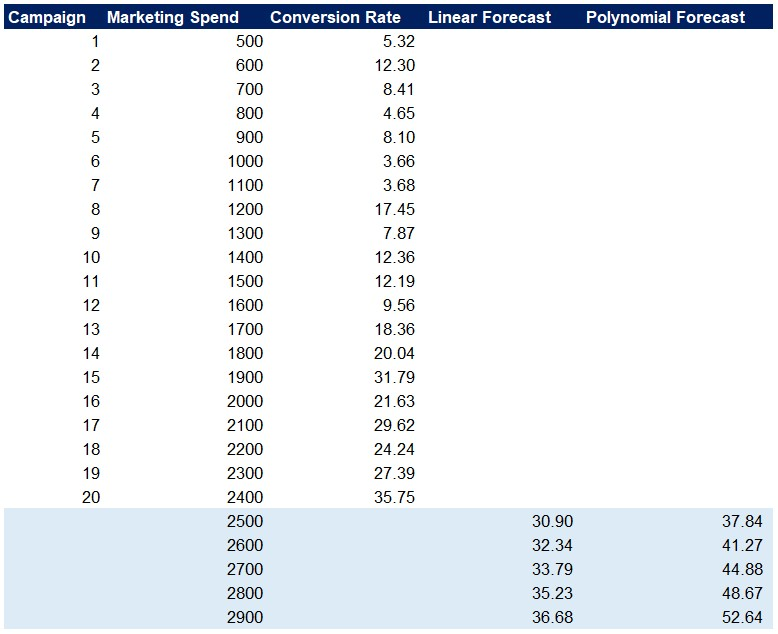Plotting these on our polynomial model chart, we get the following: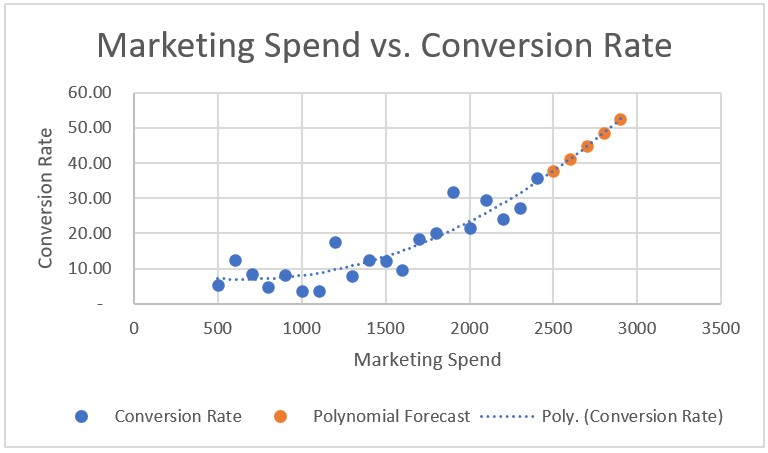We can see that the polynomial forecast seems to match the data set better when we compare the two estimates (based on the linear and polynomial regressions).## Conclusion

The polynomial regression is a great way to fit a function to a data set when we know two variables are correlated but don’t exhibit a linear relationship. We usually turn to the model whenever linear regression yields poor results and doesn’t clarify the relationship between variables.

Please, show your support by sharing the article with colleagues and friends.## FCCA, FMVA

Hi! I am a finance professional with 10+ years of experience in audit, controlling, reporting, financial analysis and modeling. I am excited to delve deep into specifics of various industries, where I can identify the best solutions for clients I work with.

In my spare time, I am into skiing, hiking and running. I am also active on Instagram and YouTube, where I try different ways to express my creative side.